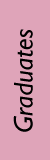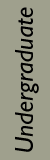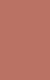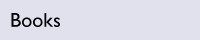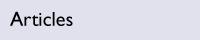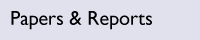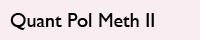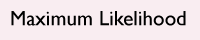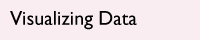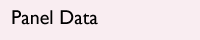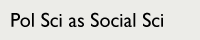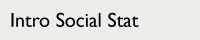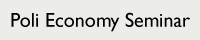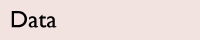Case-Based Statistics I CSSS/STAT/SOC 321 Introduces basic statistical reasoning with an emphasis on problems encountered in social science research. Measuring and summarizing data, exploratory data analysis, basic probability concepts, commonly used probability distributions, statistical inference, and basic linear regression. Focus on examples and tools. Students actively engage in statistical analysis through labs and hands-on problems.CSSS/STAT/SOC 321

Case-Based Statistics I

Offered every Fall at the
University of Washington
by various instructors

Readings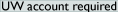Autumn 2012

Class meets:
MWF 10:30–11:20 am
Savery Hall 166

 TA: Aaron Erlich (UW Political Science)

Section meets:
T 10:30–11:20 am
Savery Hall 168

Lab meets:
Th 10:30–11:20 am
Smith Hall 220

Lectures           Click on lecture titles to view slides or the buttons to download them as PDFs.

Topic 1

Topic 2

Topic 3

Topic 4

Topic 5

Topic 6

Midterm

Topic 7

Topic 8

Topic 9

Topic 10

Topic 11

Topic 12

End of Term

Student Assignments

Problem Set 1

Due Tuesday, October 2 at the beginning of section

[5 pts. + lab credit] From Weiss (9th edition), complete problems 1.10, 1.12, 1.26, 1.42a, 1.42b, 1.52, 1.64, 2.10, 2.12, and 2.14. Also, briefly answer the following lab question: “Increasingly in international development, researchers use survey data and experiments to better understand development interventions. Choose three concepts from Chapter 1 and explain why they may be more difficult to implement in a developing country context.”

Problem Set 2

Due Tuesday, October 9 at the beginning of section

[5 pts. + lab credit] From Weiss (9th edition), complete problems 3.54, 3.62, 3.102, 3.134, and 3.152. In addition, complete the following lab assignment: Using the GSS files (or if you prefer, a suitable dataset that you have in hand for an outside project, such as a senior thesis), choose two continuous variables that have not been covered in lab. For each variable, report the five-number summary and construct a boxplot and histogram. Explain what these summaries and plots reveal about your two variables. If there are any outliers, discuss them. To receive credit on this lab assignment, submit your write-up, data visuals, and the Stata code you wrote to complete your analysis. If you do not have your own copy of Stata, be sure to budget time to complete this assignment in the lab (e.g., after your lab meeting on Thursday).

Problem Set 3

Due Tuesday, October 16 at the beginning of section

[5 pts + lab credit] From Weiss (9th edition), complete problems 14.6, 14.46, and 14.146. Then, using the data from either problem 13.74 or 13.76 (but ignoring the textbook questions for these problems), convert the provided contingency table to column percentages and explain whether the column variable appears to depend on the row variable. In addition, complete the following lab assignment, following the rules for lab assignments given above: Weiss (9th edition) problem 14.78 and your choice of problems 14.66 or 14.68.

Problem Set 4

Due Tuesday, October 23 at the beginning of section

[5 pts + lab credit] From Weiss (9th edition), complete problems 4.114, 4.131, 4.160, and 4.162. In addition, complete the following lab assignment, following the rules for lab assignments given above: Weiss (9th edition) problem 13.60.

Problem Set 5

Due Monday, November 19 at the beginning of class

[5 pts + lab credit] Read Weiss (9th edition) problem 6.100, answer the question, and then use Table II at the back of the book to calculate the percentage of boys and percentage of girls, repectively, with an FMSC score of 9.75 or less. Also from Weiss (9th edition), complete problems 5.64, 8.106, 8.116, 9.12, and 9.28. In addition, complete the following lab assignment, following the rules for lab assignments given above: Weiss (9th edition) problem 8.42.

Problem Set 6

Due Thursday, November 29 at the beginning of section

[5 pts] From Weiss (9th edition), complete problems 13.62, 13.63, 13.78, and 13.80. (There is no lab assignment this week.)

Problem Set 7

Due Tuesday, December 4 at the beginning of section

[5 pts + lab credit] Read Chapter S3 of Utts and Heckard, 2007, Mind on Statistics, provided in the electronic course reserve as s3.pdf. Then answer the following questions at the end of the chapter: S3.6, S3.7, S3.8, S3.9, S3.10, S3.12, and S3.20. In addition, complete the following lab assignment: Using the data in the Stata file CONCEPT.DTA, complete problems 11.20, 11.21, and 11.22 from the handout LastLab.pdf. Both files can be found in the electronic course reserve.

Midterm Exam

In Class, October 29 in Savery Hall 166

A review session will be held and a review sheet distributed October 26, in class.

In Class, November 16

Final Exam

December 10, 8:30 am to 10:20 am, Savery Hall 166

A review session will be held and a review sheet distributed December 7, in class.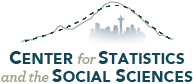Designed byChris Adolph & Erika SteiskalCopyright 2011–2022Privacy · Terms of Use